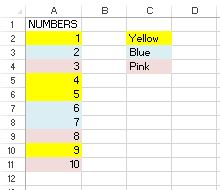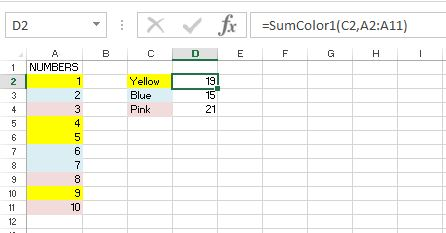# Sum By Color in ExcelWith improvements to the last few versions of Excel I see people using colors in cells more, the ability to filter by color adds to the benefit of using fill colors.  However, the increasing use of colors has led to the increasing number of requests for help I receive to sum by color in Excel.

Currently there is no function in Excel to sum by color, but you can create a UDF (User Defined Function) that will do this.  The UDF is then used similar to a standard function in Excel.

We will be using the Visual Basic for Applications (VBA) editor in Excel to create our UDF to sum by color.  If you want to read the series of posts in this area you can start from this post on Excel VBA Macros.

This post will not go into too much detail about coding, but I’m hoping that the code you’re about to use is understandable when you know what the function is doing.

# Sum By Color in Excel

The data below will be used in our demonstration on how to sum by color.

There are 10 numbers with 3 different fill colors, we will sum these cells based on their colors.## Insert Module

• Go to the VBA editor in Excel by pressing Alt and F11 together.
• Click on “Insert”
• Then “Module”We will look at a few UDFs that will sum by color in slightly different ways.

## Sum By Color Example 1

The first UDF we are going to create will be entered in cells D2 to D4.

Cells C2 to C4 have been formatted to each have one of our colors to be summed.

Our sum by color UDF will take the fill color from column C and then look for that color in column A and sum up all the cells containing that color.In the VBA editor, let’s create our first sum by color function.  I will call this function SumColor1.

Enter the lines as follows (without the numbering):

1. Function SumColor1(CellColor As Range, myRange As Range)
2. Dim clrSum As Long
3. Dim indexColor As Integer
4. indexColor = CellColor.Inter.ColorIndex
5. For Each cl In myRange
6.      If cl.Interior.ColorIndex = indexColor Then
7.           clrSum = WorksheetFunction.Sum(cl, clrSum)
8.      End If
9. Next cl
10. SumColor1 = clrSum
11. End FunctionLine 1 creates the function SumColor1 and defines two arguments, CellColor which will be the relevant cell in column C and myRange which will be the range of cells in column A.

Lines 2, 3 and 4 set up the next variables to be used.

Lines 5 to 9 contain most of our function.  Starting from the first cell in myRange we look at the cell’s fill color and if that matches the CellColor that we defined in our formula, then we include this cell in our sum function.  After this check we move onto the next cell until we have gone through all cells in myRange.

In Line 10 we set the result of the sum in 5 to 9 as the value of our SumColor1 function.

Line 11 then closes the function.

### Using SumColor1 Function

In cell D2 we enter our first SumColor1 function.

• Then we enter our first argument, the color that we want to check for and sum is the color of cell C2.
• Enter a comma “,” to move onto the second argument.
• The range that we want to check and sum by color is A2:A11.
• A closing bracket “)“ends our function.Repeat this function for D3 and D4 and you can quickly check yourself that they do work.

## Sum By Color Example 2

The last example did sum by color, but it used a cell containing a fill color to sum by that color.

Another way of doing this is to use the color index number as part of the function argument, instead of referencing a cell that has that color.

The color index for the 3 colors in the example above are Yellow = 6, Blue = 20 and Pink = 19.

How do I know these color codes?  I would like to say that I know all color codes, but the truth is I don’t.

I used a UDF to get these numbers.

### Function to get color index numbers

This is a simpler UDF with only one argument, the cell to check the color index of, we will call this function returnColor.

1. Function returnColor(myRange2 As Range)
2. returnColor = myRange2.Interior.ColorIndex
3. End FunctionI will use the cells C2 to C4 as the arguments in my functions in E2 to E4.

In E2, the formula is =returnColor(C2).This gives the color index numbers for the yellow, blue and pink fills above.

The next UDF will show you how to use a color index number instead of cell reference.

### Sum By Color With Color Index Number

The next UDF will be called SumColor2.

Instead of using a cell reference with a color to sum by, our argument will take the color index number.

1. Function SumColor2 (CellColor As Long, myRange As Range)
2. Dim clrSum As Long
3. For Each cl In myRange
4.      If cl.Interior.ColorIndex = CellColor Then
5.           clrSum = WorksheetFunction.Sum(cl, clrSum)
6.      End If
7. Next cl
8. SumColor2 = clrSum
9. End FunctionMost of the function is the same as our first example.

### Using SumColor2 Function

In F2, enter our second sum by color function as =SUMCOLOR2(6,A2:A11).

This time, instead of entering a cell to refer to a color to sum, we have entered the color index 6 which is yellow.

Our range of cells to check is A2 to A11.## Sum By Color Example 3

This 3rd example of how to sum by color in Excel will allow the user to enter the color in the function.

Instead of referencing a cell, or color index number, the user can enter “Yellow”, “Blue” or “Pink”.  We will call this function SumColor3.

1. Function SumColor3(CellColor As String, myRange As Range)
2. Dim clrSum As Long
3. Dim indexColor As Integer
4. Select Case CellColor
5. Case Is = “Yellow”
6.      indexColor = 6
7. Case Is = “Blue”
8.      indexColor = 20
9. Case Is = “Pink”
10.      indexColor = 19
11. End Select
12. For Each cl In myRange
13.      If cl.Interior.ColorIndex = indexColor Then
14.           clrSum = WorksheetFunction.Sum(cl, clrSum)
15.     End If
16. Next cl
17. SumColor3 = clrSum
18. End FunctionAgain, we can copy alot of the earlier functions we created.

Here we use Select Case to define the value of indexColor based on what is entered as the CellColor in our function’s arguments.

### Using SumColor3 Function

In G2 of our file, enter the function as follows =SumColor3(“Pink”,A2:A11).When we enter the word “Pink”, our function knows that this is color index number 19 and then checks the range from A2 to A11 for cells with this color index number as a fill, and then sums them together.

## Saving The Functions

If you tried saving your file now, you cannot save it as a standard Workbook, you must save it as a macro enabled workbook (.xlsm for 2007 and later).

If you can’t or don’t want to save your file as a .xlsm, but you have a personal workbook in Excel already, you could add these functions to it so they can be used in other files too.

If you’re not sure what a personal workbook is, or need to sum by color rarely, then maybe you could save the function to a text file and then copy into other files as and when you need them.

There are three options above to sum by color in Excel

1. Referencing a cell with the cell color to be summed
2. Using the color index number as part of the function
3. Using the color word as part of the function

Hopefully you have found this post useful, or it has at least got you thinking about creating your own functions, or what functions you would like to be able to use in Excel.